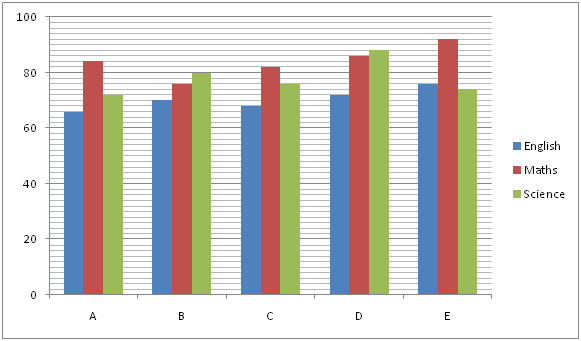# IBPS Clerk Prelims 2018 – Quantitative Aptitude Questions Day-35

Dear Readers, Bank Exam Race for the Year 2018 is already started, To enrich your preparation here we have providing new series of Practice Questions on Quantitative Aptitude – Section. Candidates those who are preparing for IBPS Clerk Prelims 2018 Exams can practice these questions daily and make your preparation effective.

[WpProQuiz 4378]

Directions (Q. 1 – 5): What value should come in place of question mark (?) in the following questions?

1) 324 × 6600 ÷ 15 % of 800 = 16 × 552 ÷ 8 +? + 286

a) 18520

b) 21360

c) 16430

d) 9540

e) None of these

2) (6240 ÷ 13) × 26 =? ÷ 9 × 54

a) 2080

b) 2560

c) 3120

d) 1750

e) None of these

3) 28 % of 2100 + 32 % of 1050 = 40 % of ? + 142

a) 1650

b) 1380

c) 2160

d) 1820

e) None of these

4) ((1224 ÷ 17 + 14 × 27) / (92 + 9)) =?

a) 8

b) 5

c) 12

d) 16

e) None of these

5) (4/8) of (8/24) of (32/64) of 324 =?

a) 27

b) 33

c) 38

d) 21

e) None of these

Directions (Q. 6 – 10) Study the following information carefully and answer the given questions:

The following bar graph shows the unit test marks of 3 subjects of 5 different students. (Out of 100)6) Find the difference between the total marks scored by Student A to that of Student C in all the given subjects?

a) 9

b) 4

c) 15

d) 22

e) None of these

7) Find the ratio between the total marks scored by Students A, C and E in English to that of the total marks scored by Students B, C and D in Science?

a) 52: 59

b) 23: 31

c) 11: 17

d) 105: 122

e) None of these

8) Find the average marks scored by all the given students in Maths?

a) 84

b) 97

c) 103

d) 115

e) None of these

9) The total marks scored by Student B in all the given subjects is approximately what percentage of total marks scored by Student E in all the given subjects?

a) 77 %

b) 84 %

c) 93 %

d) 68 %

e) 102 %

10) The total marks scored by Student A in English and Maths together is approximately what percentage more/less than the total marks scored by Student D in Maths and Science together?

a) 14 % more

b) 26 % more

c) 42 % less

d) 14 % less

e) 26 % less

Direction (1-5) :

324 × 6600 ÷ [(15/100)*800] = 16*(552/8) + x + 286

324*(6600/120) = 1104 + x + 286

17820 – 1104 – 286 = x

X = 16430

(6240 ÷ 13) × 26 = x ÷ 9 × 54

(6240/13)*26 = (x/9)*54

(6240/13)*26*(9/54) = x

X = 2080

28 % of 2100 + 32 % of 1050 = 40 % of ? + 142

(28/100)*2100 + (32/100)*1050 = (40/100)*x + 196

588 + 336 – 196 = (2/5)*x

728*(5/2) = x

X = 1820

((1224 ÷ 17 + 14 × 27) / (92 + 9)) = x

X = [(1224/17) + (14*27)] / 90

X = [72 + 378]/90 = 450/90 = 5

(4/8) of (8/24) of (32/64) of 324 = x

X = (4/8)*(8/24)*(32/64)*324 = 27

Direction (6-10) :

The total marks scored by Student A in all the given subjects

= > 66 + 84 + 72 = 222

The total marks scored by Student C in all the given subjects

= > 68 + 82 + 76 = 226

Required difference = 226 – 222 = 4

The total marks scored by Students A, C and E in English

= > 66 + 68 + 76 = 210

The total marks scored by Students B, C and D in Science

= > 80 + 76 + 88 = 244

Required ratio = 210: 244 = 105: 122

The total marks scored by all the given students in Maths

= > 84 + 76 + 82 + 86 + 92 = 420

Required average = 420/5 = 84

The total marks scored by Student B in all the given subjects

= > 70 + 76 + 80 = 226

The total marks scored by Student E in all the given subjects

= > 76 + 92 + 74 = 242

Required % = (226/242)*100 = 93 %

The total marks scored by Student A in English and Maths together

= > 66 + 84 = 150

The total marks scored by Student D in Maths and Science together

= > 86 + 88 = 174

Required % = [(174 – 150)/174]*100 = 14 % less

Kindly watch this video to know about Important Table Based Data Interpretation for IBPS Clerk Prelims 2018

Daily Practice Test Schedule | Good Luck

 Topic Daily Publishing Time Daily News Papers & Editorials 8.00 AM Current Affairs Quiz 9.00 AM Current Affairs Quiz (Hindi) 9.30 AM IBPS Clerk Prelims – Reasoning 10.00 AM IBPS Clerk Prelims – Reasoning (Hindi) 10.30 AM IBPS Clerk Prelims – Quantitative Aptitude 11.00 AM IBPS Clerk Prelims – Quantitative Aptitude (Hindi) 11.30 AM Vocabulary (Based on The Hindu) 12.00 PM IBPS PO Prelims – English Language 1.00 PM SSC Practice Questions (Reasoning/Quantitative aptitude) 2.00 PM IBPS Clerk – GK Questions 3.00 PM SSC Practice Questions (English/General Knowledge) 4.00 PM Daily Current Affairs Updates 5.00 PM Canara Bank PO Mains – Reasoning 6.00 PM Canara Bank PO Mains – Quantitative Aptitude 7.00 PM Canara Bank PO Mains – English Language 8.00 PM